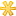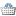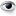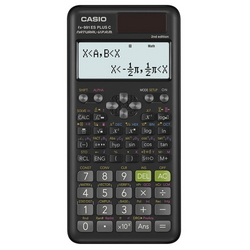BrandsShopping Cart
0 itemsRecently ViewedInformation

# CASIO Scientific Calculator Plus 2 FX-991ESClick to enlarge
 Order Code: 2090349 Model: FX-991ESPLUS-2 Unit: PC Availability: In Stock Size 77mmW x 161.5mmD x 11.1mmH Brand CASIO Color Black

Features Natural Textbook Display format for displaying equations and calculation result just as they appear in textbooks.
Two-way Power: Solar / Battery
No.
of Function: 417
Main Functions:
• Fraction calculations
• Combination and permutation
• Statistics (List-based STAT data editor, standard deviation, regression analysis)
• 9 variables
• Table function
• Comes with new slide-on hard case
• Equation calculations
• Integration/differential calculations
• Matrix calculations
• Vector calculations
• Complex number calculations
• CALC function
• SOLVE function
• Base-n calculation